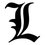# Help: Minimum

Find the value of x such that the value of $\sqrt{x²-6x+25}$ + $\sqrt{x²+10x+169}$ is at its minimum.Note by Vincent Lopez
7 months, 1 week ago

This discussion board is a place to discuss our Daily Challenges and the math and science related to those challenges. Explanations are more than just a solution — they should explain the steps and thinking strategies that you used to obtain the solution. Comments should further the discussion of math and science.

When posting on Brilliant:

• Use the emojis to react to an explanation, whether you're congratulating a job well done , or just really confused .
• Ask specific questions about the challenge or the steps in somebody's explanation. Well-posed questions can add a lot to the discussion, but posting "I don't understand!" doesn't help anyone.
• Try to contribute something new to the discussion, whether it is an extension, generalization or other idea related to the challenge.
• Stay on topic — we're all here to learn more about math and science, not to hear about your favorite get-rich-quick scheme or current world events.

MarkdownAppears as
*italics* or _italics_ italics
**bold** or __bold__ bold
- bulleted- list
• bulleted
• list
1. numbered2. list
1. numbered
2. list
Note: you must add a full line of space before and after lists for them to show up correctly
paragraph 1paragraph 2

paragraph 1

paragraph 2

[example link](https://brilliant.org)example link
> This is a quote
This is a quote
    # I indented these lines
# 4 spaces, and now they show
# up as a code block.

print "hello world"
# I indented these lines
# 4 spaces, and now they show
# up as a code block.

print "hello world"
MathAppears as
Remember to wrap math in $$ ... $$ or $ ... $ to ensure proper formatting.
2 \times 3 $2 \times 3$
2^{34} $2^{34}$
a_{i-1} $a_{i-1}$
\frac{2}{3} $\frac{2}{3}$
\sqrt{2} $\sqrt{2}$
\sum_{i=1}^3 $\sum_{i=1}^3$
\sin \theta $\sin \theta$
\boxed{123} $\boxed{123}$

## Comments

Sort by:

Top Newest

$x=1$

- 7 months, 1 week ago

Log in to reply

Mind sharing your solution bro? I'm kinda stuck with this

$\sqrt{x²-6x+9+16}$ + $\sqrt {x²+10x+25+144}$

=$\sqrt{(x-3)²+4²}$ + $\sqrt{(x+5)²+12²}$

I know solving the x-intercept of the line passing through the points $(3,-4)$ and $(-5,12)$ or $(3,4)$ and $(-5,-12)$ will give the answer but I don't know why is it like that.

- 7 months, 1 week ago

Log in to reply

i used calculus for this; idk the algebraic way. If you want to know the calculus route i can share it

- 7 months, 1 week ago

Log in to reply

Yes bro, if you don't mind.

- 7 months, 1 week ago

Log in to reply

You can find the derivative of the function and set that equal to 0. Then solve for $x$. To make sure that it is a minimum point, take the second derivative of the function to see whether it is concave up on the stationary point (concave up means that $\displaystyle \frac{d^2 y}{dx^2} > 0$ )

- 7 months, 1 week ago

Log in to reply

$f(x)=\sqrt{x²-6x+25} +\sqrt{x²+10x+169}$

$f'(x)=\frac{x-3}{\sqrt{x²-6x+25}} +\frac{x+5}{\sqrt{x²+10x+169}}=0$

$\frac{x+5}{\sqrt{x²+10x+169}}=-\frac{x-3}{\sqrt{x²-6x+25}}$

Squaring both sides of the equation

$\frac{(x+5)²}{x²+10x+169}=\frac{(3-x)²}{x²-6x+25}$

The reciprocals of equals are equal

$\frac{x²+10x+169}{(x+5)²}=\frac{x²-6x+25}{(3-x)²}$

$\frac{(x+5)²+12²}{(x+5)²}=\frac{(x-3)²+4²}{(3-x)²}$

$1+\frac{12²}{(x+5)²}=1+\frac{4²}{(3-x)²}$

$\frac{12²}{(x+5)²}=\frac{4²}{(3-x)²}$

Get the square root of both sides

$\frac{12}{x+5}=\frac{4}{3-x}$

$\boxed{x=1}$

Is this the right way bro?

- 7 months, 1 week ago

Log in to reply

yeah. Don't forget to check if it is a minimum point with the second derivative!

- 7 months, 1 week ago

Log in to reply

When I thought I was done but completely forgotten the most important part, my eyes can't keep up anymore. I'll check it tomorrow bro, thanks for helping me again :)

- 7 months, 1 week ago

Log in to reply

haha no problem!

- 7 months, 1 week ago

Log in to reply

$f(x)=\sqrt{x²-6x+25} +\sqrt{x²+10x+169}$

$f'(x)=\frac{x-3}{\sqrt{x²-6x+25}} +\frac{x+5}{\sqrt{x²+10x+169}}$

$f''(x)=\frac{16}{\sqrt{x²-6x+25}} +\frac{144}{\sqrt{x²+10x+169}}$

I don't quite get it bro, do I need find out if $f''(1)>0$?

- 7 months, 1 week ago

Log in to reply

yes

- 7 months, 1 week ago

Log in to reply

Thanks bro for helping me all throughout the solution :) I don't even know how to use emoji. hahaha

- 7 months, 1 week ago

Log in to reply

lol no problem mate

- 7 months, 1 week ago

Log in to reply

I think you are almost done. Let $A(3,-4), B(-5,12), P(x,0)$, then $AP+BP \geq AB$ by triangle inequality, and the equality holds when $P$ lies on segment $AB$.

- 7 months, 1 week ago

Log in to reply

@X X Why is it that point A has an ordinate of $-4$ instead of $+4$?

- 7 months, 1 week ago

Log in to reply

If the y coordinate is 4, the distance from P to A won't change. However, P cannot be on segment AB, so the triangle inequality's equality won't hold.(i.e. Choose A and B such that A and B are on different side of the X axis

- 7 months, 1 week ago

Log in to reply

@X X Took me long to understand, thanks man. :)

- 7 months, 1 week ago

Log in to reply

$-\infty?$ @Vincent Lopez

- 7 months, 1 week ago

Log in to reply

@Percy Jackson it's 1 but I don't know how to solve it.

P.S edited already, should be help.

- 7 months, 1 week ago

Log in to reply

×

Problem Loading...

Note Loading...

Set Loading...